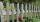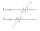# Division + line segment - math problems

#### Number of problems found: 7

• Divide in ratioLine segment AB 12 cm long divide in a ratio of 5: 3. How long are the individual parts?
• My fatherMy father cut 78 slats on the fence. The shortest of them was 97 cm long, the longer one was 102 cm long. What was the total length of the slats in cm?
• HexagonDivide a regular hexagon into lines into nine completely identical parts; none of them must be in a mirror image (individual parts can only be rotated arbitrarily).
• Points on line segmentPoints P & Q belong to segment AB. If AB=a, AP = 2PQ = 2QB, find the distance: between point A and the midpoint of the segment QB.
• Line segmentCut a line segment of 15 cm into two line segments so that their lengths are in ratio 2:1. What length will each have?
• DividingDivide the three line segment 13 cm, 26 cm and 19.5 cm long for parts so that the individual parts were equally long and longest. How long will the individual parts and how many it will?
• Line segmentLine segment AB is 8 cm long. Divide it in a ratio of 2: 3.

We apologize, but in this category are not a lot of examples.
Do you have an interesting mathematical word problem that you can't solve it? Submit a math problem, and we can try to solve it.

We will send a solution to your e-mail address. Solved examples are also published here. Please enter the e-mail correctly and check whether you don't have a full mailbox.

Please do not submit problems from current active competitions such as Mathematical Olympiad, correspondence seminars etc...